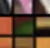# 1. 何为 Neural Style Transfer

Neural Style Transfer 指的是给定一张内容图片（Content，以下简称 C）和一张风格图片（Style，以下简称 S），将 S 图片中的风格迁移到 C 图片上，生成一张新的图片（Generated，以下简称 G），如图 1 所示（其中的生成图片来自 Justion Johnson）。

# 2. 深度卷积网络学到了什么

要实现 Neural Style Transfer，首先需要理解卷积网络中各层所提取的特征，也就是网络中各层都学到了些什么。

假设你训练了一个如图 2 所示的卷积网络，可以通过下述方法对网络中各层的计算内容进行可视化。在网络计算整个训练集的过程中，在网络中的第一层上选择一个隐藏单元，找到能使该单元输出的激活值最大的 9 个输入图像，如图 2 所示。由于一个隐藏单元只能看到整个网络的一小部分，该隐藏单元的输入图像只是很小一块。

由图 2 可以看到，该隐藏单元对从左上角到右下角的斜线会产生很大的激活值，即该隐藏单元会检测图片中从左上到右下的斜线。

选择 9 个隐藏单元重复以上步骤，得到结果如图 3。可见每个隐藏单元都有自己的检测目标。通过图 3，可以看出第 1 层中的隐藏单元都在搜索简单的特征，比如边界或不同颜色的色块。

对于网络中更深处的层，其中的隐藏单元能看到更大部分的图像。从第 2 层中选择 9 个隐藏单元，找到使每个单元输出最大激活值的 9 张图像，如图 4 所示。可以看到，第 2 层的隐藏单元检测的内容更复杂，开始检测各种特定的纹理。

在第 3 层上进行同样的操作，得到结果如图 5。可见第 3 层中的隐藏单元开始检测图样和部分物体，如网格、人的上半身等。

在第 4 层上得到的结果如图 6，开始识别特定目标，如左上角表示的隐藏单元在识别狗的头部，注意这些狗看上去都很像。

在第 5 层上得到的结果如图 7，可以看到识别的内容更加复杂了，如右下角表示的隐藏单元也在检测狗，但这里的狗外表各异，它检测的是更加广泛的狗的概念。

以上例子都来自这篇论文，其中使用了更复杂的方式对卷积神经网络进行可视化。

# 3. 代价函数

对于 Neural Style Transfer 的任务，对于生成图像 G ，我们希望其内容与图像 C 接近，并且希望其风格和图像 S 接近。可以定义 Neural Style Transfer 的代价函数为：

\begin{equation}
J(G) = \alpha J_{content}(C, G) + \beta J_{style}(S, G) \tag{1}
\end{equation}

式 (1) 中，$J_{content}$ 为内容代价函数，$J_{content}(C, G)$ 衡量了 C 和 G 在内容上的相似程度；$J_{style}$ 为风格代价函数，$J_{style}(S, G)$ 衡量了 S 和 G 在风格上的相似程度。$\alpha$ 和 $\beta$ 是两个超参数，用于控制在内容和风格上的相似程度。虽然这里使用一个超参数就可以达到相同的效果，但算法的原作者使用了两个超参数，这里以原作者的实现为准。

生成图像 G 的方法如下：

1. 随机初始化图像 G，假设其大小为 $100 \times 100 \times 3$；
2. 使用梯度下降最小化代价函数 $J(G)$，在这个过程中，要更新的是图像 G 的像素值，而不再是权重等参数；

举例来说，对于如图 8 左图的内容图像 C 和如图 8 右图的风格图像 S：

首先随机初始化图像 G，然后随着最小化 $J(G)$ 的过程，图像 G 中的各个像素被不断更新，其内容变得越来越像 C 图像，风格越来越像 S 图像，如图 9。

以上算法来自这篇论文

# 4. 内容代价函数

前面提到，Neural Style Transfer 的代价函数由内容代价函数和风格代价函数组成，其中内容代价函数的计算方式如下：

1. 选择一个隐藏层 $l$ 用于计算内容代价；
2. 选择一个已经预训练过的卷积网络，如 VGG 网络；
3. 计算 C 和 G 在该网络第 $l$ 层上的激活值，分别记为 $a^{[l](C)}$ 和 $a^{[l](G)}$；
4. 如果 $a^{[l](C)}$ 和 $a^{[l](G)}$ 相似，则认为 C 和 G 具有相似的内容，即计算：

\begin{equation}
J_{content}(C, G) = \frac{1}{2} \left\vert a^{[l](C)} – a^{[l](G)} \right\vert_2^2 \tag{2}
\end{equation}

式 (2) 中 $a^{[l](C)}$ 和 $a^{[l](G)}$ 是两个向量，二者逐元素相减，并计算相减结果的 L2 范数的平方。开头的 $\frac{1}{2}$ 是一个归一化常量。

最小化式 (2) 的过程，相当于寻找合适的 G，使得 $J_{content}(C, G)$ 值最小，即寻找与图像 C 的内容最相似的图像 G。

需要注意的是，在第 1 步中，一般会选取网络中间的某层用于计算内容代价。由于较浅的层只会识别一些低级特征，选取这些层会导致图像 C 和 G 过于相似，如要求 G 和 C 在像素级别上都是一致的；又由于较深的层会识别一些高层概念，选取这些层会导致 C 和 G 差异过大，如 C 中有一条狗，则只要 G 中也有一条狗，则认为 C 和 G 一样。

# 5. 风格代价函数

要定义风格代价函数，首先需要定义如何计算“风格”。一张图像的风格可以使用如下的方式来计算：将图像输入一个卷积网络，选择其中第 $l$ 层来计算，则图像的风格可以定义为该层激活值的各个通道间的相关（Correlation）。

例如对于如图 10 的网络，选择其中红框所示的一层来计算风格，将该层的激活值取出来，如图 11 所示。

图 11 中为激活值的每个通道染上了不同的颜色，这里为了方便展示，假设该层激活值只有 5 个通道，实际中通道数会更多。假设该层中各通道检测的内容如如 12 所示，第 1 个通道（图 11 中红色通道）计算的是垂直线条的问题（图 12 中红色方框），第 2 个通道（图 11 中黄色通道）计算的是橙色色块（图 12 中黄色方框）。通过计算第 1 个通道和第 2 个通道之间的相关值可以得到两个通道间的相关程度，如果两个通道相关程度高，则表示输入在输入图像中，如果某一个位置出现了垂直线条（通道 1 检测的特征），则在该位置很可能也会出现橙色色块（通道 2 检测的特征）；反之，如果两个通道的相关程度低，则在图像的同一位置上不太可能同时出现垂直线条和橙色色块。

通过计算两个通道的激活值的相关，我们可以知道这两个通道所检测的图像特征是否可能会在一个位置同时出现。二者相关的程度，即相关值的大小，可以用来衡量不同图像特征在同一位置出现的频率。

具体来说，对于给定的图像，使用风格矩阵（Style Matrix）表示其风格。记第 $l$ 层位于 $(i, j, k)$ 位置上的激活值为 $a_{i,j,k}^{[l]}$，其中 $i$、$k$、$k$ 分别表示位置的高度、宽度、通道数。记风格矩阵为 $G^{[l]}$，它是一个 $n_c^{[l]} \times n_c^{[l]}$ 的矩阵，$n_c^{[l]}$ 为第 $l$ 层的通道数，则 $G_{k, k’}^{[l]}$ 表示第 $k$ 通道和第 $k’$ 通道激活值的相关值，其计算方式为：

\begin{equation}
G_{k, k’}^{[l]} = \sum_{i=1}^{n_h^{[l]}} \sum_{j=1}^{n_w^{[l]}} a_{i,j,k}^{[l]} a_{i,j,k’}^{[l]} \tag{3}
\end{equation}

式 (3) 中，$n_h^{[l]}$ 和 $n_w^{[l]}$ 分别表示第 $l$ 层激活值的高度和宽度，即对于第 $l$ 层激活值中的第 $k$ 和 $k’$ 通道，遍历这两个通道的各个位置，逐元素相乘并求和。如果 $k$ 和 $k’$ 两个通道的激活值相关程度高，则 $G_{k, k’}^{[l]}$ 的值较大。注意虽然之前一直说的是“相关”，式 (3) 实际上计算的是非归一化的互协方差（Corss Covariance）。另外要注意式 (3) 中的 $G$ 指的不是生成图像的 G，它指的是格拉姆矩阵（Gram Matrix）。

使用式 (3) 分别计算风格图像 S 和生成图像 G 的风格矩阵 $G_{k, k’}^{[l](S)}$ 和 $G_{k, k’}^{[l](G)}$，由此可以定义风格代价函数如下：

\begin{align}
J_{style}^{[l]} &= \frac{1}{(2 n_h^{[l]} n_h^{[l]} n_c^{[l]})^2} \left \Vert G^{[l](S)} – G^{[l](G)} \right \Vert_F^2 \\
&= \frac{1}{(2 n_h^{[l]} n_h^{[l]} n_c^{[l]})^2} \sum_k \sum_{k’} (G^{[l](S)} – G^{[l](G)})^2 \tag{4}
\end{align}

式 (4) 计算的是 $G^{[l](S)}$ 和 $G^{[l](G)}$ 两个矩阵之间的 Frobenius 范数的平方，并在开头乘以了一个归一化常数。实际计算中这个归一化常数并不重要，因为这个代价函数还会再乘上某个超参数。